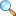# Linear Algebra: Important Definitions and Results

These notes are made and shared by Mr. Akhtar Abbas. We are really very thankful to him for providing these notes and appreciates his efforts to publish these notes on MathCity.org. These notes contains important definitions with examples and related theorem, which might be helpful to prepare interviews or any other written test after graduation like PPSC, FPSC or etc.

 Name Linear Algebra: Important Definitions and Results Mr. Akhtar Abbas 22 pages PDF (see Software section for PDF Reader) 560 kB

### Contents & Summary

• If a vector space $V$ has a basis with $n$ elements, then $n$ is called dimension of $V$.
• If $W$ is a subspace of $V$, then dim(W) = dim(V).
• The span of column of a matrix $A$ is called column space of $A$.
• The span of rows of a matrix $A$ is called row space of $A$.

Please click on View Online to see inside the PDF.

• Download PDF (560.33 KiB, 2917 downloads) |View Online